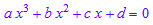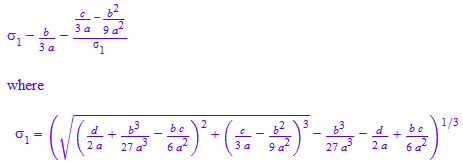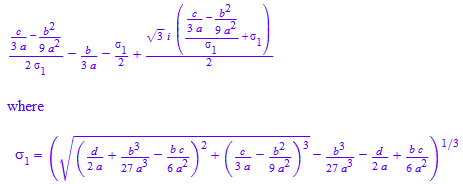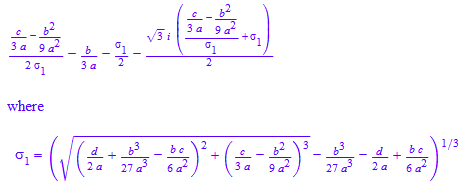## Monday, August 13, 2012

You can use the following formula to Solve 3rd order Polynomial Equation.
Ifthen its root x(1),x(2),x(3) are as fallow

x1=x2=x3=